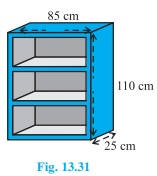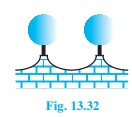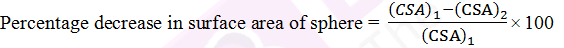### NCERT Solutions For Class 9 Math Chapter – 13 Exercise – 13.9

1. A wooden bookshelf has external dimensions as follows: Height = 110cm, Depth = 25cm,

Breadth = 85cm (see fig. 13.31). The thickness of the plank is 5cm everywhere. The external faces are to be polished and the inner faces are to be painted. If the rate of polishing is 20 paise per cm2 and the rate of painting is 10 paise per cm2, find the total expenses required for polishing and painting the surface of the bookshelf.Solution:

External dimensions of book self,

Length, l = 85cm

Height,

h = 110 cm

External surface area of shelf while leaving out the front face of the shelf

= lh+2(lb+bh)

= [85×110+2(85×25+25×110)] = (9350+9750) = 19100

External surface area of shelf is 19100 cm2

Area of front face = [85×110-75×100+2(75×5)] = 1850+750

So, area is 2600 cm2

Area to be polished = (19100+2600) cm= 21700 cm2.

Cost of polishing 1 cm2 area = Rs 0.20

Cost of polishing 21700 cm2 area Rs. (21700×0.20) = Rs 4340

Dimensions of row of the book shelf

Length(l) = 75 cm

Breadth (b) = 20 cm and

Height(h) = 30 cm

Area to be painted in one row= 2(l+h)b+lh = [2(75+30)× 20+75×30] = (4200+2250) = 6450

So, area is 6450 cm2.

Area to be painted in 3 rows = (3×6450)cm= 19350 cm2.

Cost of painting 1 cm2 area = Rs. 0.10

Cost of painting 19350 cm2 area = Rs (19350 x 0.1) = Rs 1935

Total expense required for polishing and painting = Rs. (4340+1935) = Rs. 6275

Answer: The cost for polishing and painting the surface of the book shelf is Rs. 6275.

2. The front compound wall of a house is decorated by wooden spheres of diameter 21 cm, placed on small supports as shown in fig. 13.32. Eight such spheres are used forth is purpose, and are to be painted silver. Each support is a cylinder of radius 1.5cm and height 7cm and is to be painted black. Find the cost of paint required if silver paint costs 25 paise per cm2 and black paint costs 5 paise per cm2.Solution:

Diameter of wooden sphere = 21 cm

Radius of wooden sphere, r = diameter/ 2 = (21/2) cm = 10.5 cm

Formula: Surface area of wooden sphere = 4πr2

= 4×(22/7)×(10.5)= 1386

So, surface area is 1386 cm3

Radius of the circular end of cylindrical support = 1.5 cm

Height of cylindrical support = 7 cm

Curved surface area = 2πrh

= 2×(22/7)×1.5×7 = 66

So, CSA is 66 cm2

Now,

Area of the circular end of cylindrical support = πr2

= (22/7)×1.52

= 7.07

Area of the circular end is 7.07 cm2

Again,

Area to be painted silver = [8 ×(1386-7.07)] = 8×1378.93 = 11031.44

Area to be painted is 11031.44 cm2

Cost for painting with silver colour = Rs(11031.44×0.25) =Rs 2757.86

Area to be painted black = (8×66) cm2 = 528 cm2

Cost for painting with black colour =Rs (528×0.05) = Rs26.40

Therefore, the total painting cost is:

= Rs(2757.86 +26.40)

= Rs 2784.26

3. The diameter of a sphere is decreased by 25%. By what percent does its curved surface area decrease?

Solution:

Let the diameter of the sphere be “d”.

Radius of sphere, r1 = d/2

New radius of sphere, say r2 = (d/2)×(1-25/100) = 3d/8

Curved surface area of sphere, (CSA)1 = 4πr12 = 4π×(d/2)2 = πd2 …(1)

Curved surface area of sphere when radius is decreased (CSA)= 4πr22 = 4π×(3d/8)2 = (9/16)πd2 …(2)

From equation (1) and (2), we have

Decrease in surface area of sphere = (CSA)1 – (CSA)2

= πd– (9/16)πd2

= (7/16)πd2= (7d2/16d2)×100 = 700/16 = 43.75% .

Therefore, the percentage decrease in the surface area of the sphere is 43.75% .